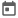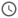# Verilog Vectors##### 03 Aug 2021##### 3 mins

In Verilog we have seen that we have 1-bit data types. But in hardware, many ports can have more than one bit has an input. Also, it can have registers which is nothing but a group of flip flops. Thus, Verilog provides us a way to use both single bit variables and multiple bits variable.

### Scalar variables

Any `reg` or `wire` declaration, that has only one bit is known as scalar variables.

### Vector variables

Any `reg` or `wire` declaration that has more than one bit is known as vector variables. The range is specified using `[msb:lsb]` or `[lsb:msb]` brackets just after writing the data type.

#### Vector Slicing

Any subpart of the vectors can be selected, and this is known as vector slicing. After declaring a vector, subpart can be selected using the `[]` brackets and providing the range in between.
Vector slicing should be in the same order as it is declared. It means that the if during declaration msb is written first then, during slicing should be done from higher bits to lower bits and vice-versa.

Syntax for vector slicing:
`[<higer-bit>:<lower_bit>]` or
`[<lower_bit>:<higer-bit>]`

A disadvantage of this method is that we can't have a variable as a slicing parameter. Slicing parameter should always be constant. So, Verilog provides a one more way to slice vectors, in which we can use variables as parameter. Verilog provides `+:` and `-:` operators for slicing.

Syntax for using this slicing operator:
`[<starting_bit>+:<width>]`
`[<starting_bit>-:<width>]`

#### Example

``````module vector_slicing;
reg [7:0] var1;     // reg variable having width of 8
reg [31:0] var2;    // reg variable having width of 32
reg [0:15] var3;    // reg variable having width of 16
reg [3:0] temp;     // reg variable having width of 4

initial begin
var1 = 8'b100xx100;
var2 = 32'ha34cd125;
var3 = 16'h78ff;

\$display("var1 is %b", var1);
\$display("var2 is %h", var2);
\$display("var3 is %h", var3);

var1 = 1; // 4th bit of var1 is set to 1
\$display("var1 is %b", var1);

temp = var2[3:0]; // selecting the first 4 bits of var2
\$display("temp is %b", temp);

temp = var3[3:0]; // not valid
\$display("temp is %b", temp);

temp = var3[10+:4];
\$display("temp is %h", temp);

temp = var2[7+:4];
\$display("temp is %h", temp);

// temp = var2[(7*i):(7*i+4)]; // not valid

temp = var2[(8*i)+:4]; // var2[16+:4]
\$display("temp is %h", temp);

temp = var2[(8*i)-1-:4]; // var2[15-:4]
\$display("temp is %h", temp);
end
endmodule``````